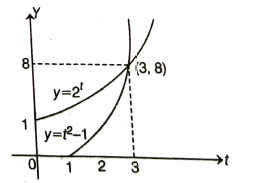Trigonometric equations
Question

# A solution of the equation $\left(1-\mathrm{tan}\mathrm{\theta }\right)\left(1+\mathrm{tan}\mathrm{\theta }\right){\mathrm{sec}}^{2}\mathrm{\theta }+{2}^{{\mathrm{tan}}^{2}\mathrm{\theta }}=0$ which $\theta$ lies in the interval $\left(-\mathrm{\pi }/2,\mathrm{\pi }/2\right)$ is given by

Difficult
Solution

## By inspection (or by graph) we findGet Instant Solutions